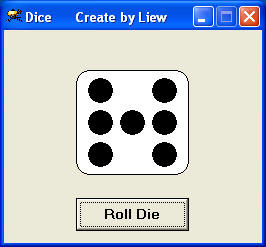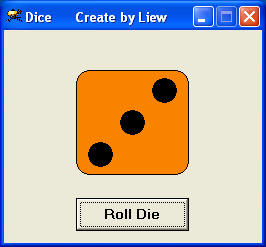# Dice with Different Colors

This program creates a dice that displays different colors on different faces. It can be used to play board games when you cannot find a physical dice. You may incorporate it into any VB games that require a dice. Some of the VB games that you can create in Visual Basic are snakes and ladders game, monopoly and more.

In this program, we use the Randomize function Rnd to generate random numbers. Rnd function will produce random numbers between 0 and 1. Multiplying Rnd with 6 will then generate random numbers between 0 and 6 , therefore 1+Rnd*6 will produce numbers between 0 and 7, and Int(1+Rnd*6) will then generate random integers from 1 to 6 because Int is a function that return the integer part of the number. For example, Rnd=0.4852, 6*Rnd=2.9112, 1+6*RND=3.9112, so INT(1+6*Rnd)=INT(3.9112)=3.

To produce different colors, we use the color codes in VB which you can get it from the color palette in the VB properties window. The syntax to assign certain color to an object  is object.FillColor=color code.

Color codes in VB take the form of hexadecimal numbers such as hc010f.

By creating shape(n) control  array and make them appear according to a generated random number you can then have a perfect virtual dice that is even better than a physical dice!The Run Time Interface### The Code

```Private Sub Command1_Click()
Dim n as integer
Randomize Timer
n = Int(1 + Rnd * 6)
For i = 0 To 6
Shape1(i).Visible = False
Next

If n = 1 Then
Shape1(3).Visible = True
Shape2.FillColor = &hc0c0c0

End If
If n = 2 Then
Shape1(2).Visible = True
Shape1(4).Visible = True
Shape2.FillColor = &h8080ff
End If

If n = 3 Then
Shape1(2).Visible = True
Shape1(3).Visible = True
Shape1(4).Visible = True
Shape2.FillColor = &h80ff&
End If

If n = 4 Then
Shape1(0).Visible = True
Shape1(2).Visible = True
Shape1(4).Visible = True
Shape1(6).Visible = True
Shape2.FillColor = &hffff00
End If

If n = 5 Then
Shape1(0).Visible = True
Shape1(2).Visible = True
Shape1(3).Visible = True
Shape1(4).Visible = True
Shape1(6).Visible = True
Shape2.FillColor = &hffff&
End If

If n = 6 Then
Shape1(0).Visible = True
Shape1(1).Visible = True
Shape1(2).Visible = True
Shape1(4).Visible = True
Shape1(5).Visible = True
Shape1(6).Visible = True
Shape2.FillColor = &hff00ff
End If
End Sub
```